## PHP关于foreach的问题

```<?php
\$a = array('abe','ben','cam');
foreach (\$a as \$k=>&\$n)
\$n = strtoupper(\$n);
foreach (\$a as \$k=>\$n) // notice NO reference here!
echo "\$n\n";
print_r(\$a);
?>

will result in:

ABE
BEN
BEN
Array
(
 => ABE
 => BEN
 => BEN
)```

`Reference of a \$value and the last array element remain even after the foreach loop. It is recommended to destroy it by unset().`

0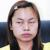0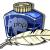\$a = array('abe','ben','cam');
foreach (\$a as \$k=>&\$n)
\$n = strtoupper(\$n);
foreach (\$a as \$k=>\$n) // notice NO reference here!
print_r(\$a);

Array
(
 => ABE
 => BEN
 => ABE
)
Array
(
 => ABE
 => BEN
 => BEN
)
Array
(
 => ABE
 => BEN
 => BEN
)

0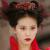&\$n的值已经是一个引用了。

0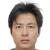```foreach (\$a as \$k=>\$a) { // notice NO reference here!
echo "\$n\n";
}```

foreach内部执行：

\$a = \$a  //\$a = ‘ABE’
\$a = \$a  //\$a = ‘BEN’
\$a = \$a //\$a = ‘BEN’

0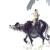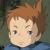0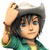0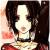```<?php
\$a = array('abe','ben','cam');
// array to upcase
foreach (\$a as \$k=>&\$n)
\$n = strtoupper(\$n);
print_r(\$a);

// do someting else
// now we use a temp var named \$n
\$n = 'some else';
print_r(\$a);

?>

// print 1
Array
(
 => ABE
 => BEN
 => CAM
)

// print 2
Array
(
 => ABE
 => BEN
 => some else   // have been changed
)```0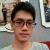```\$a = array('abe','ben','cam');
foreach (\$a as \$k=>&\$n){
\$n = strtoupper(\$n);
}
foreach (\$a as \$k=>\$n) {
//由于\$n在上一次遍历时已经被定义为了引用，所以这里虽然你没有声明引用，但\$n变量仍然为一个引用，它指向的目标就是上一次遍历时最后的那个元素,因此，本次遍历时，其实每次都是在修改\$a的最后一个元素而已。

//第一次将\$n修改为了\$a;
//ABE,BEN,ABE
//第二次将\$n修改为了\$a;
//ABE,BEN,BEN
//第三次将\$n修改为了\$a;
//ABE,BEN,BEN
echo "\$n\n";
}
print_r(\$a);```